# Inter maths 1A chapter 6 exercise 6(a) solutions

Intermediate mathematics 1st year chapter 6 Trigonometric Ratios upto Transformations textbook solutions for exercise 6(a).

These solutions are very easy to understand. Study the text boo lesson very well.

Observe the example problems and solutions in the text book. Try them.

Observe the given solutions and try them in your own method.

You can also see

Inter Maths 1A textbook solutions

Inter Maths 1B textbook solutions

Inter Maths IIA textbook solutions

Inter Maths IIB textbook solutions

Trigonometric Ratios upto Transformations

Exercise 6(a)

Exercise 6(b)

Exercise 6(c)

Exercise 6(d)

Exercise 6(e)

Exercise 6(f)

Inter Maths Trigonometry text book solutions

M

## Chapter 6 – Trigonometric Ratios upto Transformations

Exercise 6(a)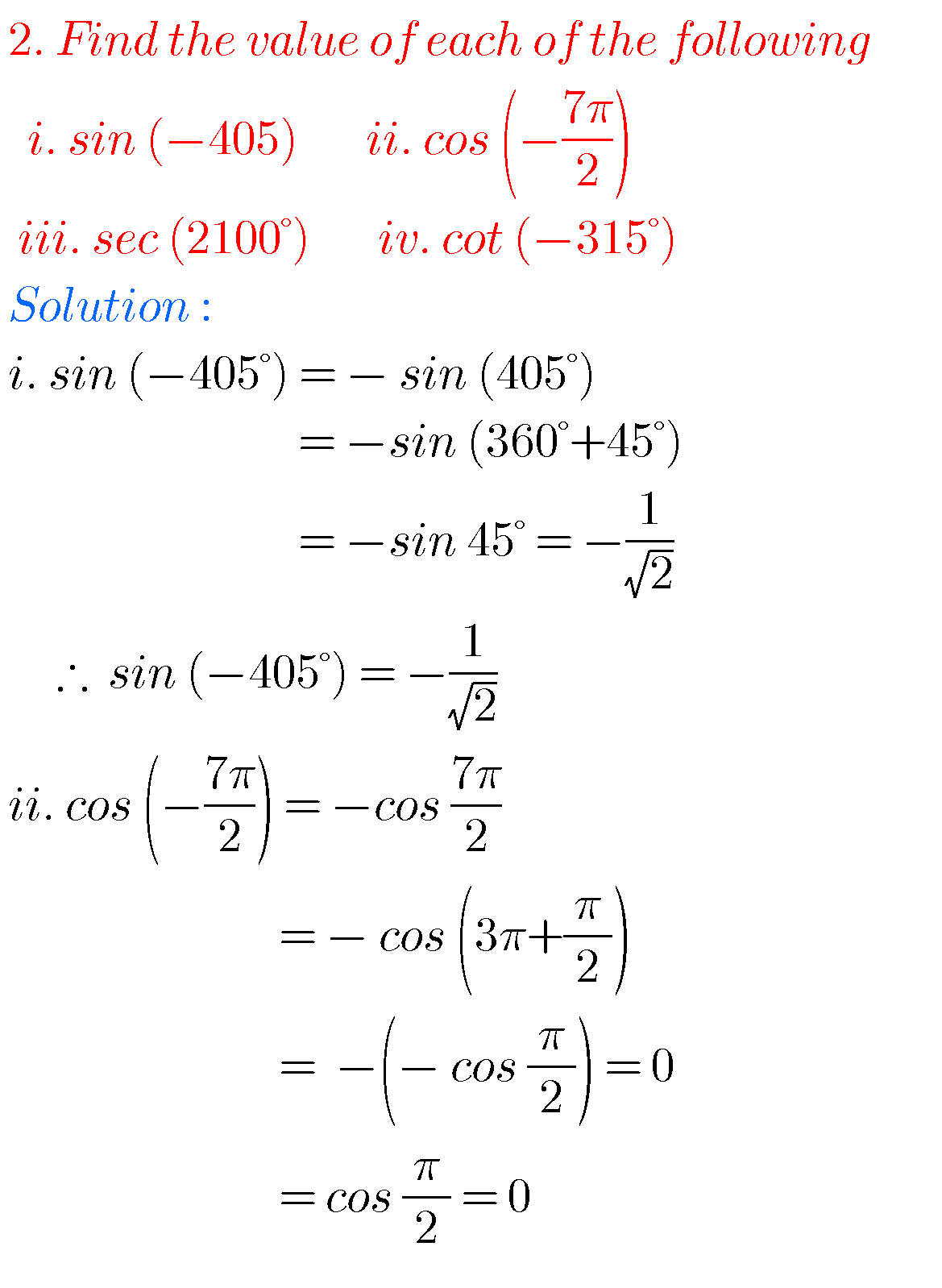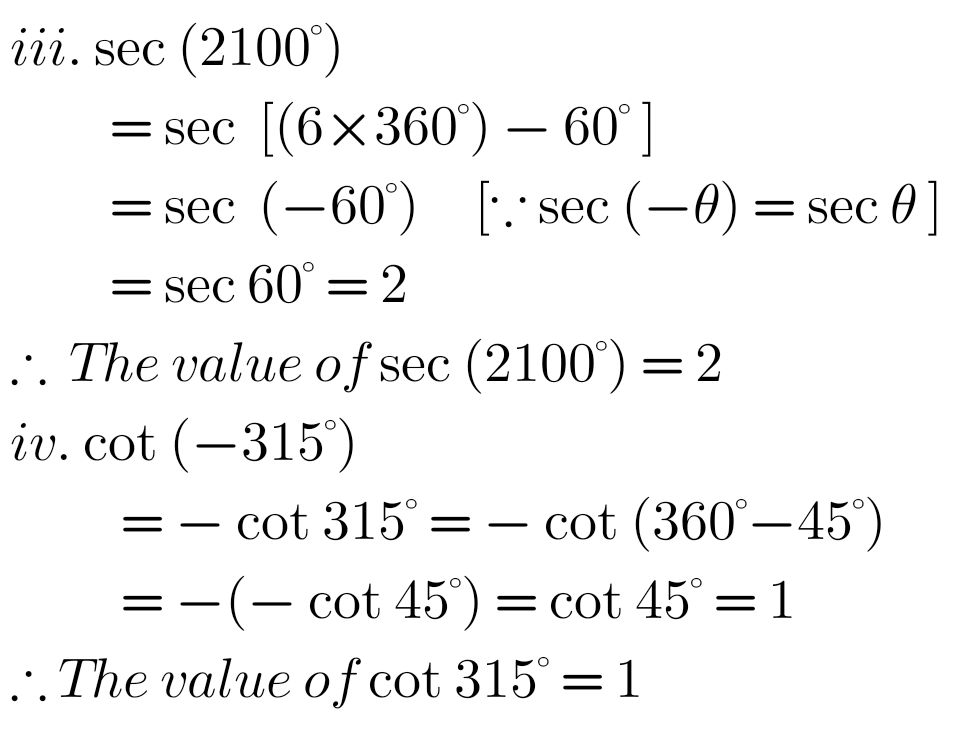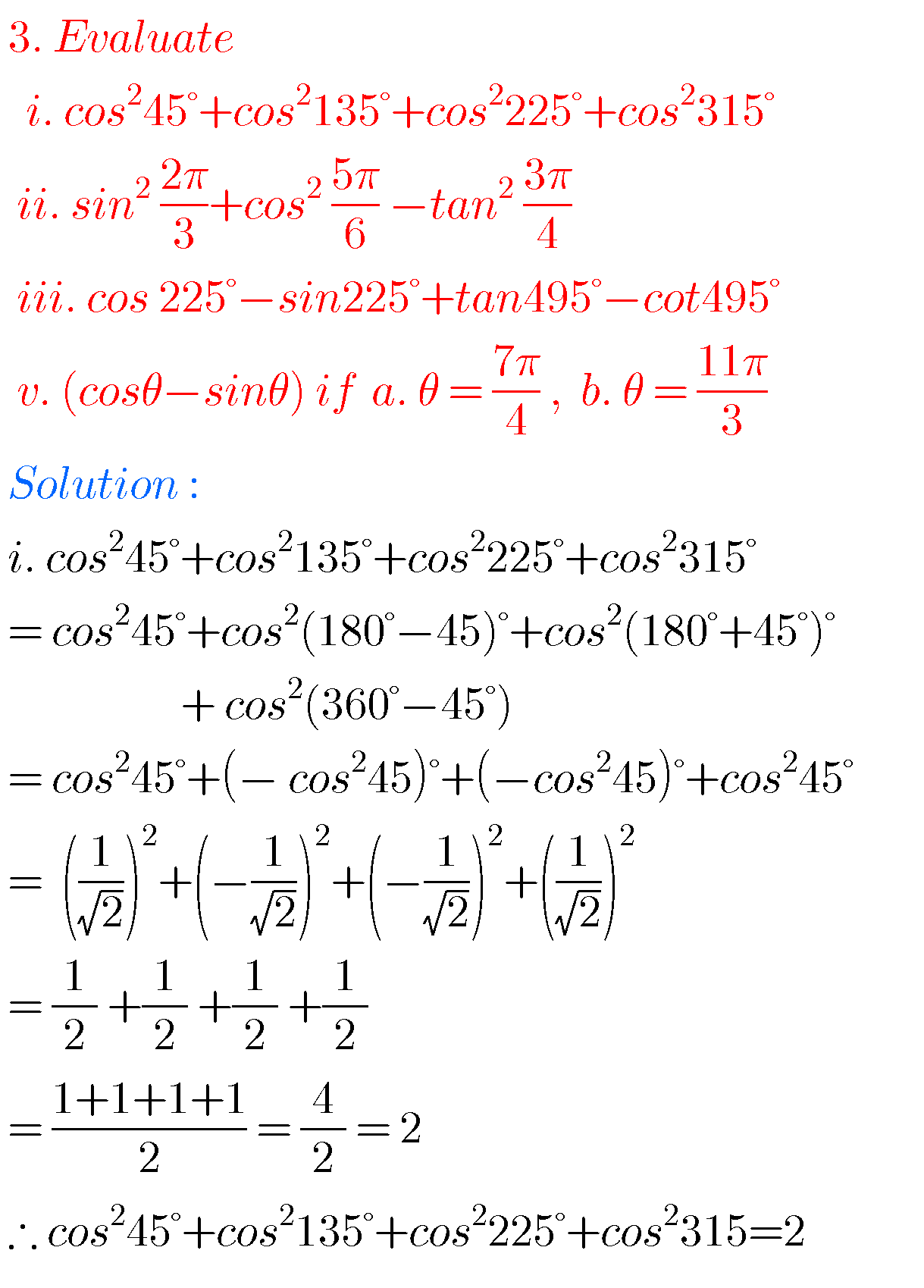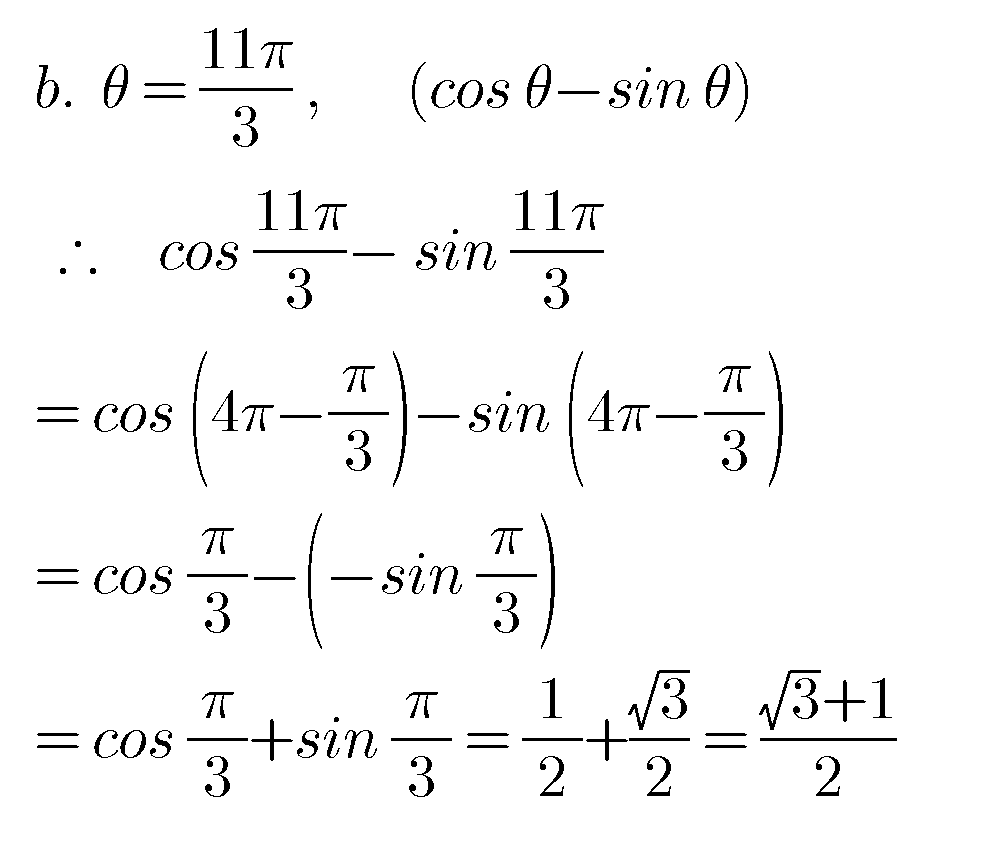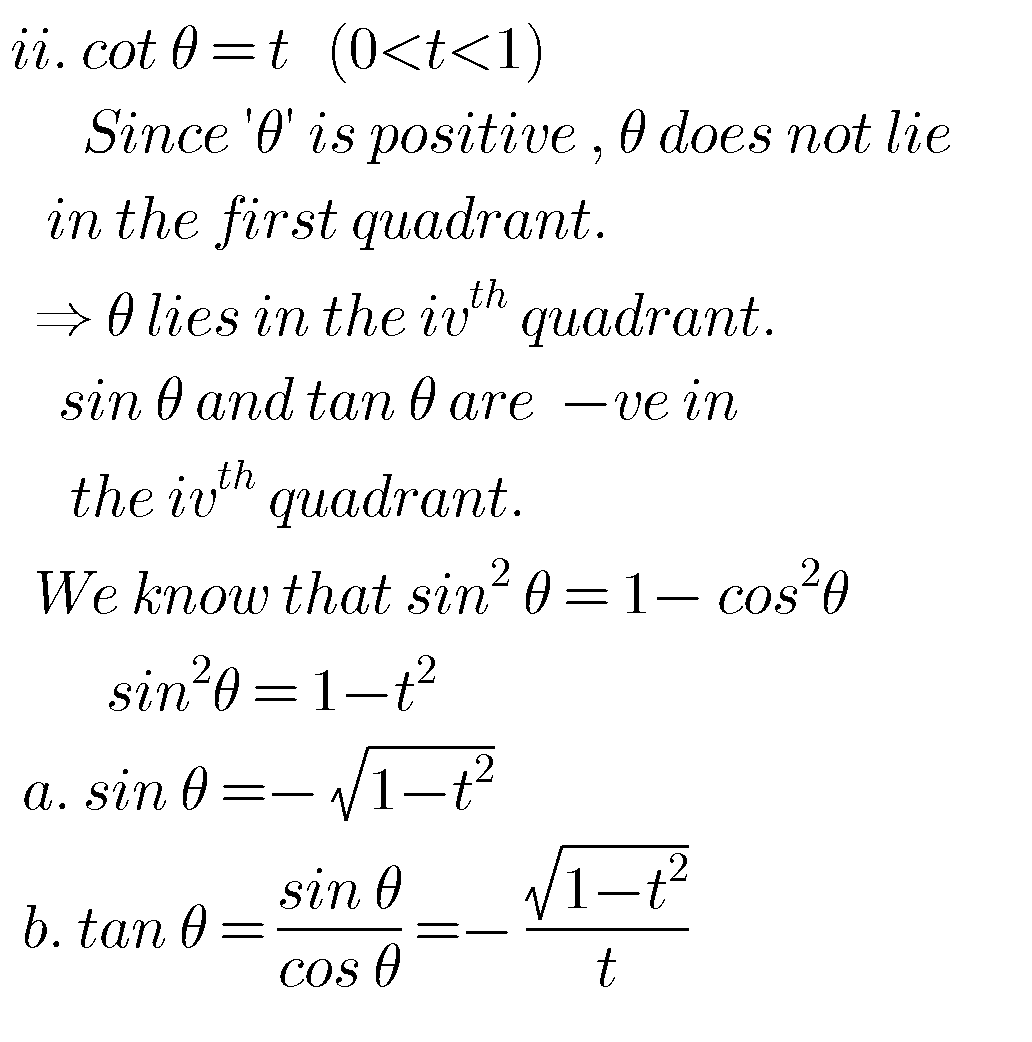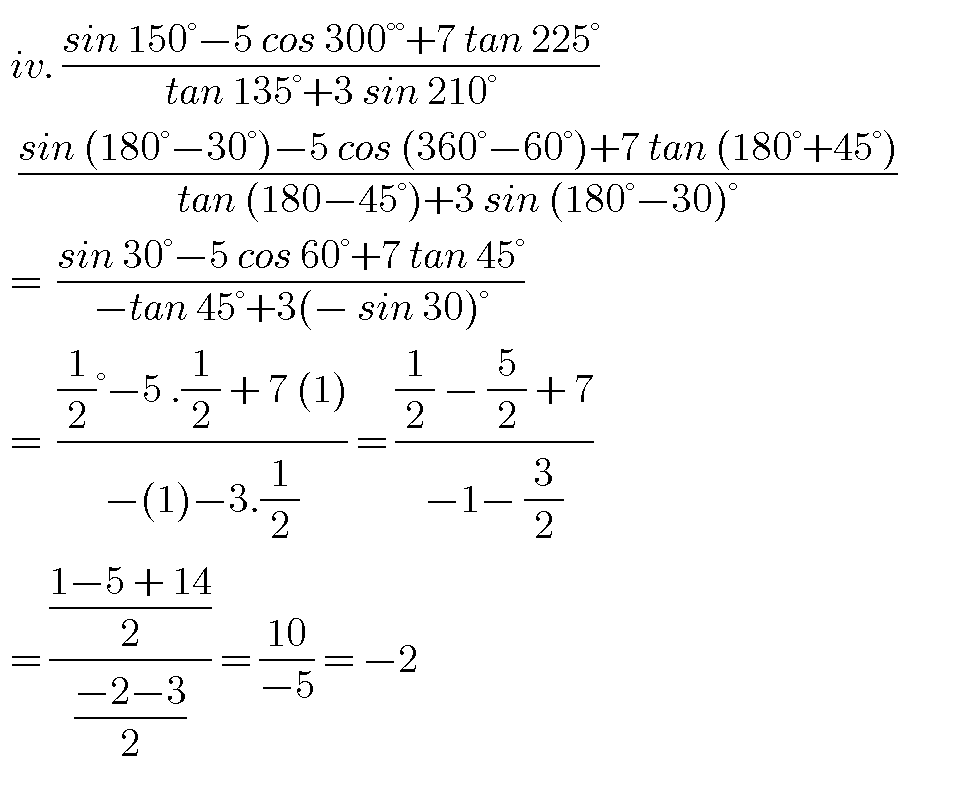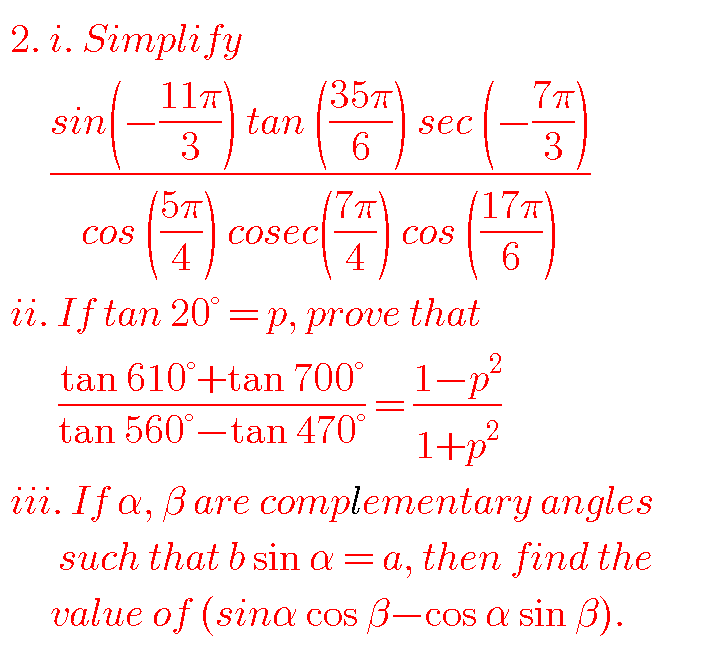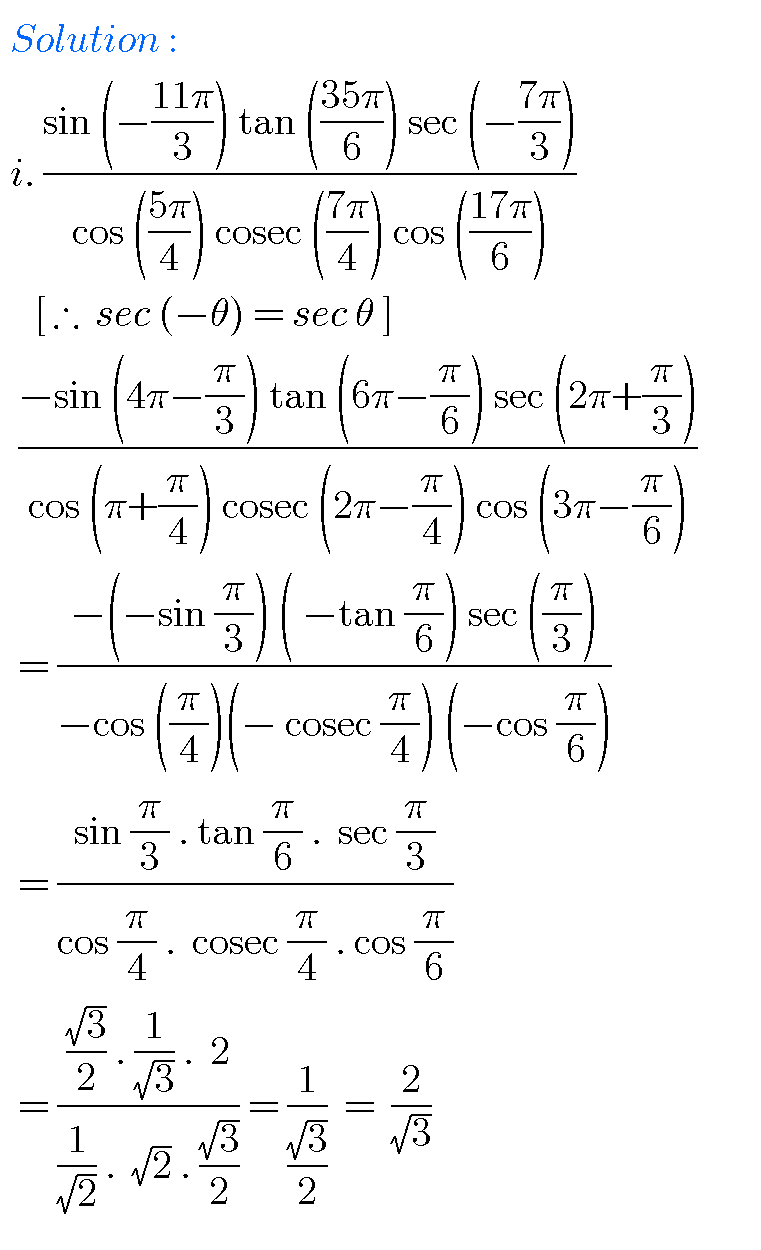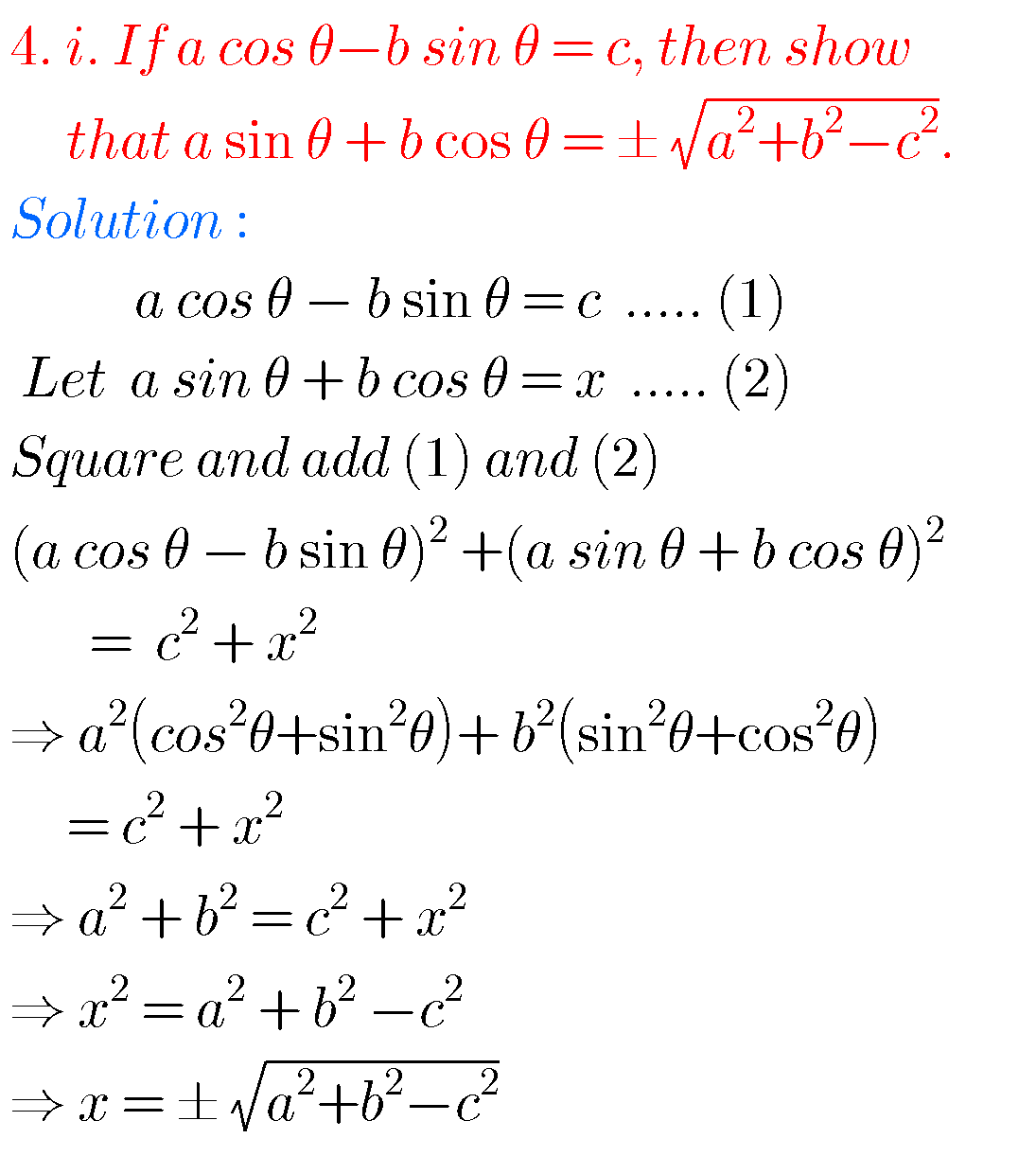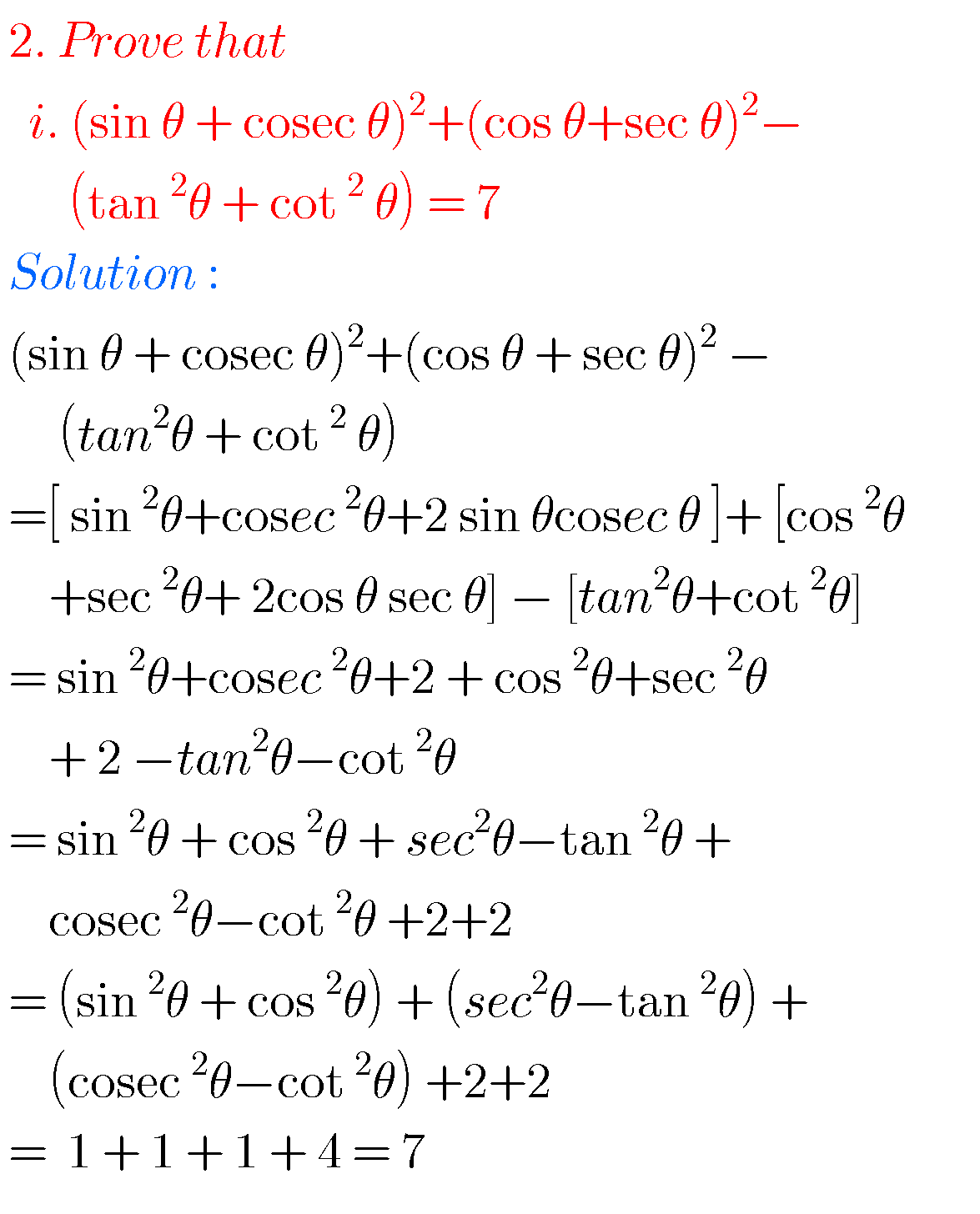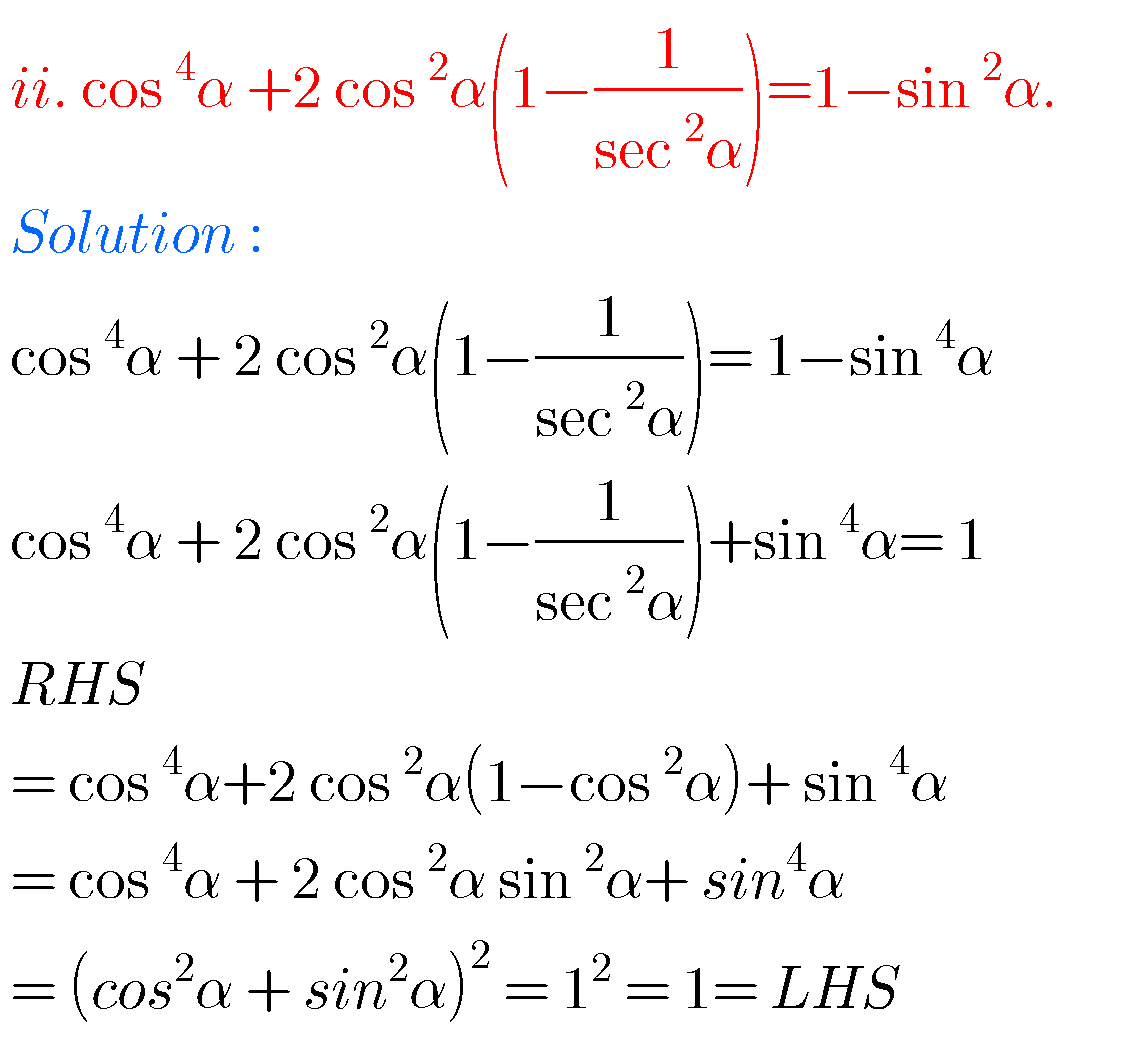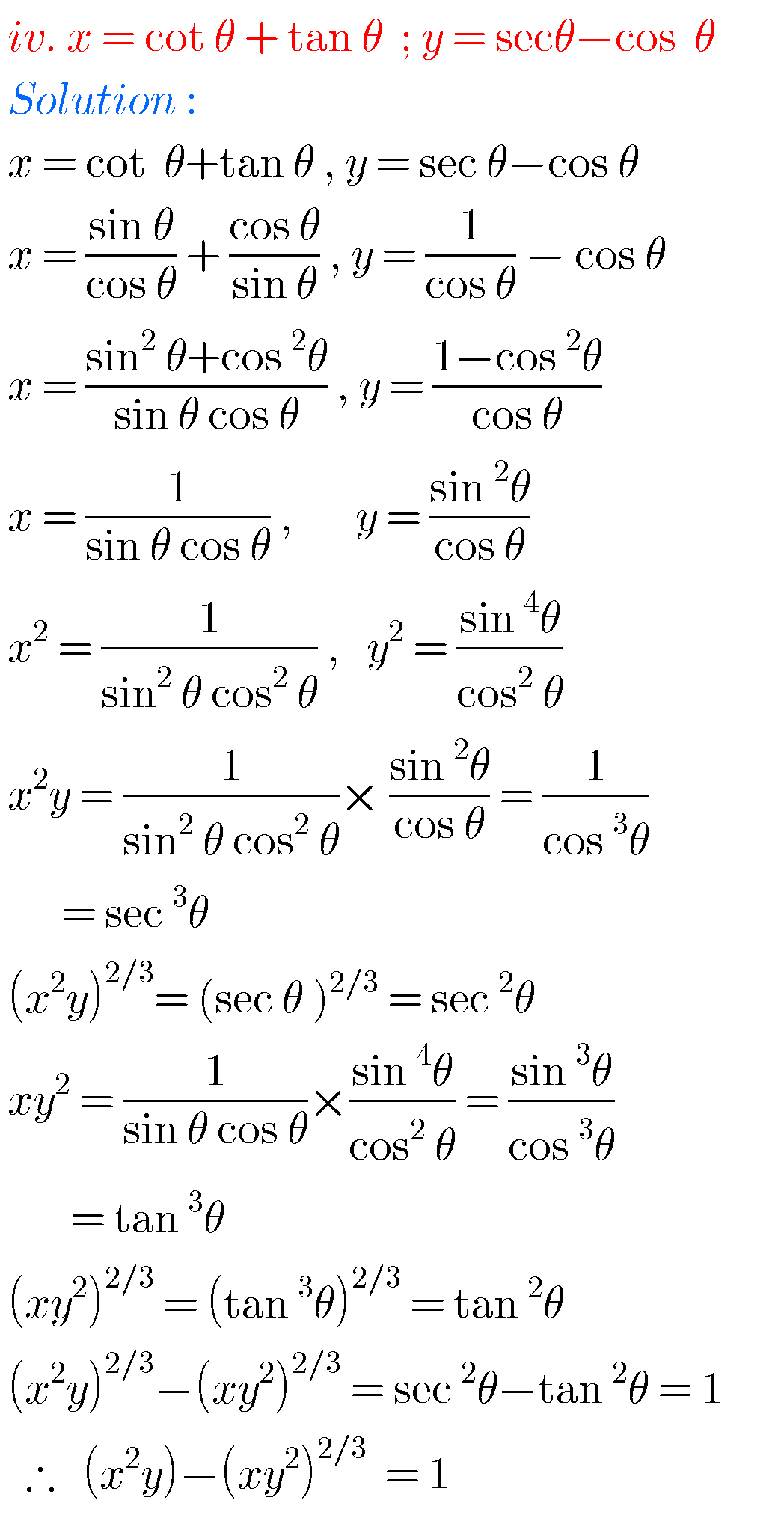Note :Observe the solutions and try them in your own methods.

Some moreYou can also see the solutions for Inter maths for examination purpose.

Maths 1A

3. Matrices

Ncert maths class 6 chapter 14 solutions

Nios maths 311 book 1 Sets 1.1 solutions

Class 10 solutions for real numbers

Class 10 solutions for sets

### 3 thoughts on “1st Inter exercise 6(a) Solutions maths 1A ,chapter 6 Trigonometric Ratios upto Transformations”

1.Tq

2.Tq very much

3.Thank you 😊